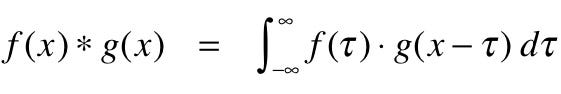Understanding Convolutional Neural Networks(CNNs)

CNN’s has a large application in the field of image processing and video processing. Let’s understand how CNN’s works.

So in this article, I will cover all the details about how Convolutional Neural Networks evolved and why they are so good with images. We will also be having a hands-on session on which we will build a Convolutional Neural Network using Keras. So let’s get started.

What is Convolutional Neural Networks(CNNs) ?

Convolutional Neural Networks are similar to ordinary neural networks. They are also made up of neurons and learn the weights and biases. These networks work best with images, they take images as input and then encode certain properties in the architecture.

The name “convolutional neural networks” indicates that the network employs a mathematical operation called the convolution.

Convolution is an operation of two functions of real-valued arguments.

In convolutional neural network terminology, the first argument to the convolution is often referred to as input and the second argument as the kernel and the output of them is called the feature map.

Now I will show how you can apply this mathematical term “convolution” in our CNN. So let’s move on to that.

So as you can see the matrix in green is the input(a matrix made up of pixels of the input image) and the matrix in yellow is the kernel. So here you can see how the kernel matrix convolves with the input matrix to give us a feature map. But wait!! you can see some change in the dimensions of the feature map. Don’t worry we will cover it in details after a while. Let’s move on to the next topic which is Pooling.

Pooling Layer

Pooling layer is mostly used in between consecutive convolutional layer. It is used to reduce the spatial size of the representation to reduce the number of parameters and the computation of the network. The pooling layer is applied independently to every depth slice of the input and it reduces the spatial dimensions of the input. It is mostly used to reduce over-fitting. If we apply a MAX POOLING on an input with a filter size 2X2 and stride of 2 then it will down-sample the input size by a factor of 2 in both width and height keeping the depth unaffected which means it discarded 75% of the activation. Now below is an image which contains how a pooling layer is implemented.

Now we will talk about the formula which we use in calculating the dimensions of the output layer.

In this formula, p and s are padding and striding respectively. We will get into the details of it one by one.

Padding is used to add extra pixels around the edges. Actually what Padding does is that it makes sure that the pixels at the corner to get the needed attention. By attention, I mean that in convolution when the kernel strides around the input matrix, the pixels at the middle gets more weight as it appears more than once in convolution operation while the corner pixels are involved in only one convolution operation. So padding gives an extra layer or more around the original matrix so that the corner pixels are considered.

Striding

Striding in convolutional neural network is very important. I will discuss here how to striding is implemented with the help of two images to get it clear.

So in this image, we can see that the instead of sending the red box by only one step we are taking a jump or two. One main reason for using a larger stride is to reduce the number of parameters in the output feature map.

Now we are ready to design our own CNN model. I will explain one layer of CNN in details so that you can get a grip on it.

Designing a Convolutional Neural Network

In this part, we are going to design our own Convolutional Neural Network. CNN’s consists of convolutional layer, pooling layer, and fully connected layer at the end (We can add softmax at the end for a multiclass problem).

The architecture which we will use is given in the image below. I will be implementing using Keras. As I want this article to be small and precise, maybe someday I will code a CNN from scratch. For now, let’s get into the architecture. So we will be implementing a two-layered Convolutional Neural Network and I have used ReLU activation function and max-pooling technique. There are two fully connected layers with a softmax activation at the end.

``model = Sequential()``
``model.add(Conv2D(32, kernel_size=(5, 5), strides=(1, 1),                 activation='relu',                 input_shape=input_shape))model.add(MaxPooling2D(pool_size=(2, 2), strides=(2, 2)))model.add(Conv2D(64, (5, 5), activation='relu'))model.add(MaxPooling2D(pool_size=(2, 2)))model.add(Flatten())model.add(Dense(1000, activation='relu'))model.add(Dense(num_classes, activation='softmax'))``
``model.compile(loss=keras.losses.categorical_crossentropy,              optimizer=keras.optimizers.SGD(lr=0.01),              metrics=['accuracy'])``
``model.fit(x_train, y_train,          batch_size=batch_size,          epochs=epochs,          verbose=1,          validation_data=(x_test, y_test),          callbacks=[history])``

In the first layer, we have used 32 filters of size 5X5 with stride 1 and ReLU activation function. Next, we have added a max pooling layer. In the layer we have used 64 filters of size 5X5, followed by a max pooling layer. Then we used a flattened layer. After that, we have used two dense layers with ReLU and softmax activations respectively. Then we used cross-entropy as our loss function and stochastic gradient descent(SGD) to minimize the loss. Then we train the model according to our use case.

So you saw how easy it is to code a CNN using Keras. Please try to implement using a data-set of your own.

Hope you enjoyed this article. I will be writing another article on “Visualizing Convolutional Neural Networks” and that will be interesting because I will show how activations at each layer contribute to learning certain features.

If you have any suggestions mail me at subham.tiwari186@gmail.com or join me on Twitter

Source: Deep Learning on Medium